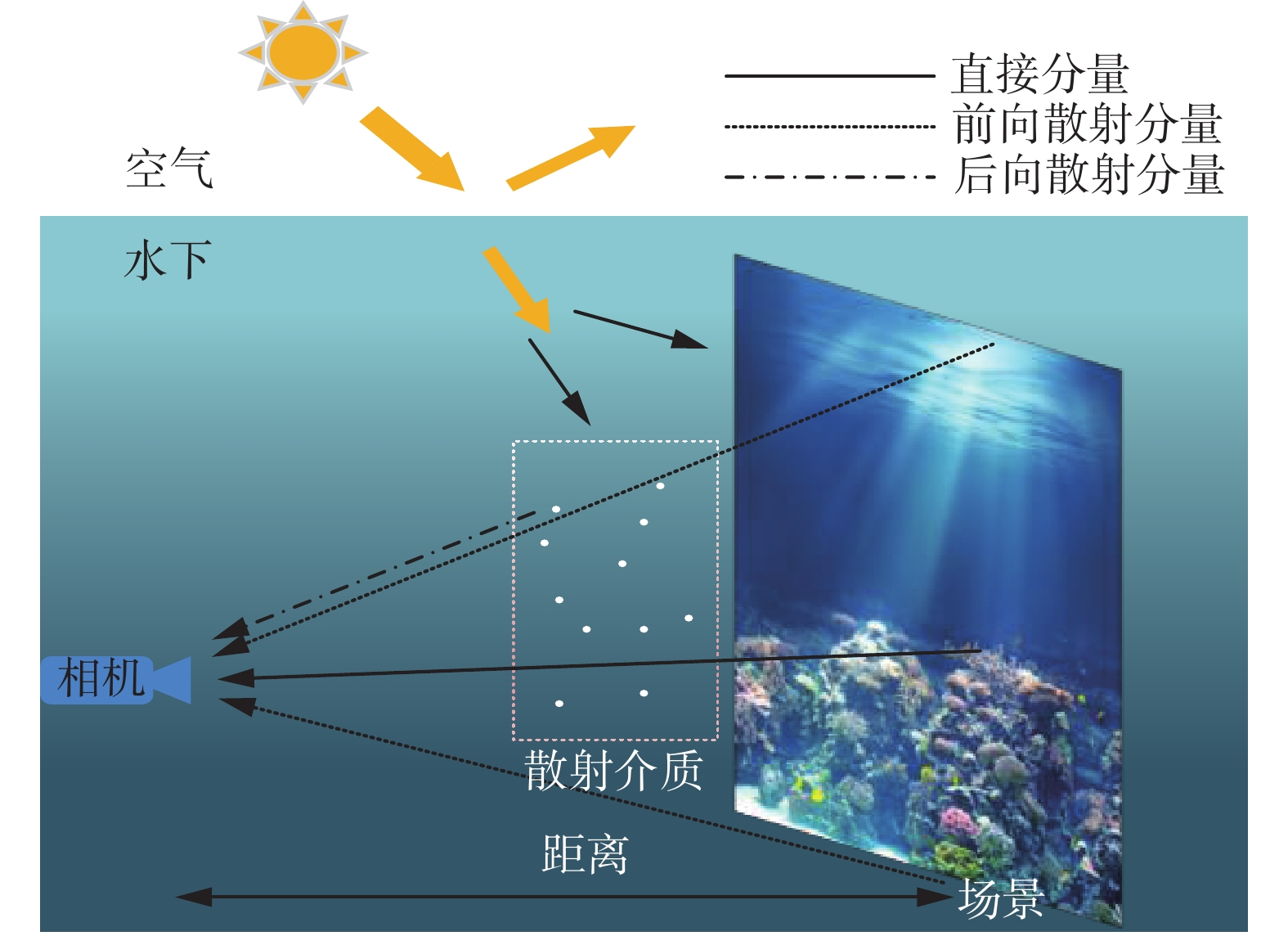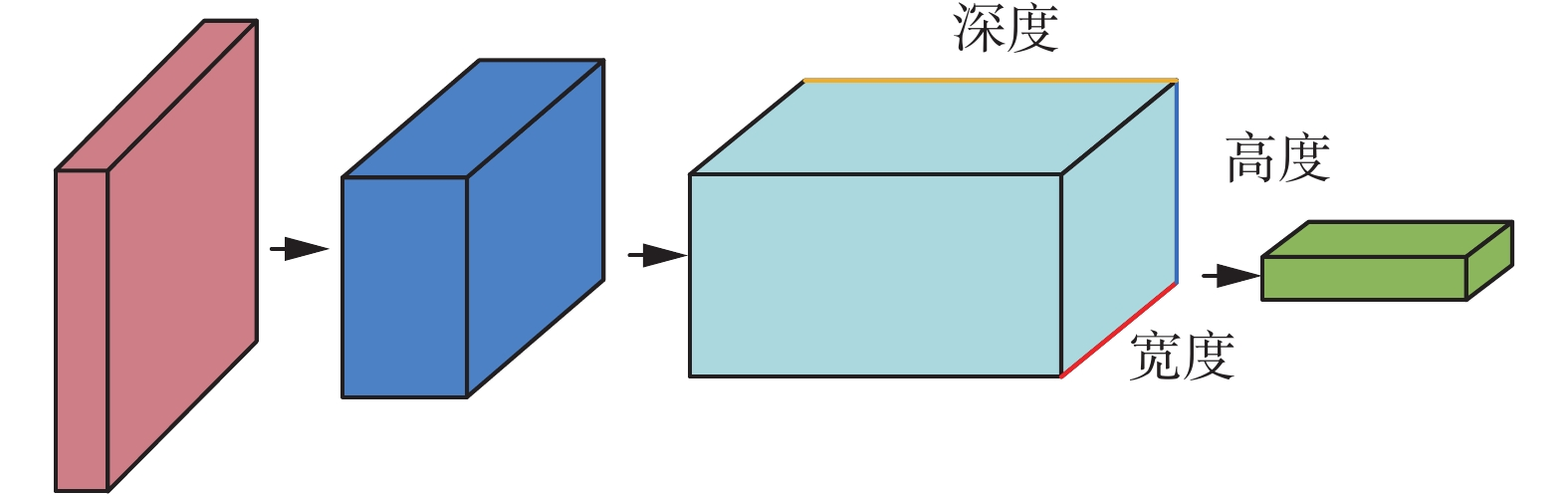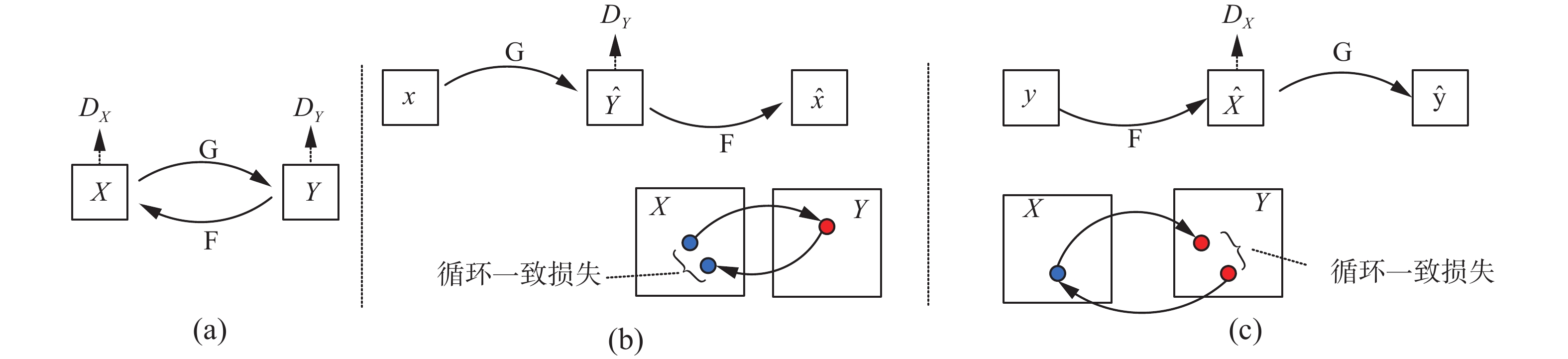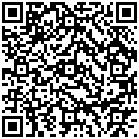引用本文: 严浙平, 曲思瑜, 邢文. 水下图像增强方法研究综述 [J]. 智能系统学报, 2022, 17(5): 860-873.YAN Zheping, QU Siyu, XING Wen. An overview of underwater image enhancement methods [J]. CAAI Transactions on Intelligent Systems, 2022, 17(5): 860-873.
 Citation: YAN Zheping, QU Siyu, XING Wen. An overview of underwater image enhancement methods [J]. CAAI Transactions on Intelligent Systems, 2022, 17(5): 860-873.## 水下图像增强方法研究综述

##### doi: 10.11992/tis.202108022

###### 通讯作者: 邢文. E-mail： xingwen@hrbeu.edu.cn.
• 中图分类号: TP391.41; TN911.73

## An overview of underwater image enhancement methods

• 摘要: 水下图像增强是水下有人/无人设备完成深海探测任务的重要支撑技术。该技术综合应用信号处理、图像处理以及机器学习的相关理论知识以实现对水下图像的灵活增强。在简述了水下图像增强的研究背景、意义及热点问题的基础上，按照不基于成像模型、基于成像模型与基于学习3个方向对水下图像增强技术的发展进行了详细的论述，重点分析了不同方法的原理和技术特点。最后，根据水下图像增强技术的难点与目前面临的主要问题对研究方向和发展趋势进行了归纳和展望。

Abstract: The underwater image enhancement technology plays an important role in deep-sea exploration missions for underwater manned/unmanned vehicles, which adopts relevant and comprehensive theoretical knowledge of signal processing, image processing, and machine learning to realize the flexible enhancement of underwater images. In this paper, a brief description of the research background, significance, and the hotspot of underwater image enhancement is provided first. Then, the underwater image enhancement methods are expounded, considering the image-formation-model-free, image-formation-model-based, and learning-based approaches. Particularly, the design principles and technical characteristics of different methods are analyzed. Finally, considering the shortcomings of the existing methods and recent major challenges of the underwater image enhancement technology, the future research direction and development trend of this technology are summarized and prospected.

• 在陆地空间和资源压力日益增大的条件下，对于水下空间的开发变得迫切起来，海底蕴藏着丰富的矿产资源与化石能源，能掌握高效的水下资源开发技术的国家必将在未来发展中占尽先机。高质量且清晰的水下图像是海洋资源勘探和海洋安全监测等一系列水下作业任务的重要保证。但是，水下成像是不同于陆地上的成像机制，水下环境的成像是更为复杂的。首先，光线在水中的传播衰减与陆地的传播衰减是不同的，它是一种不均匀的且依赖于波长特性的衰减。红光是可见光中波长最长的，在传播时，红光最先消失，然后是按照波长顺序的橙光、绿光及蓝光，这也是大多数水下的图像都呈现出蓝绿色调的原因。其次，水体中的溶解物也会对成像造成影响，水体中的粒子对于光有反射作用，当其反射的光到达相机时会对所成的像产生散射效果，散射使成像的细节变得模糊影响图像的质量。不同深度、位置、季节、气温对于水体的影响是十分复杂的，加大了去除图像中粒子散射效果的难度。最后，当达到一定的水深时，太阳光无法到达提供照明，必须引入辅助照明设备进行拍摄，此时，图像中心区域会不可避免的出现亮斑，严重影响了图像的对比度。由于光线的选择性衰减与水中粒子散射问题，水下图像会表现出颜色扭曲、细节模糊、对比度低的问题。

早期的水下图像处理是应用硬件设备辅助进行的，如偏振器法[1-2]，该方法基于偏振器的原理实现了去除散射效应的目的。在偏振器后提出了用快速快门门限成像方法来去除水下图像的散射或噪声，该方法利用具有超高快门速度的成像设备快速地打开和关闭快门实现了对散射光线的去除。Martin等提出了立体成像先验水下图像增强方法，使用两个摄像机以不同的角度对同一场景进行拍摄，进而建立起场景的立体结构，由于水下图像的衰减与场景的立体结构是有关联的，因此可降低场景成像的模糊程度。这些应用硬件的方法普遍存在效率低、成本高、无法用于深水、难携带等弊端，难以满足现代海洋调查的需求。

随着图像处理技术的快速发展，利用光学成像进行水下探测展现出巨大的潜力和优势。由于不同水体类型的光吸收特性、折射特性、透明度等参数具有显著差异性，设计能够在多种复杂条件下对水下图像进行增强的算法是非常重要的。国内外研究学者相继提出了许多新颖的算法和研究思路，本文将对不基于物理模型、基于物理模型与基于学习的3类水下增强算法进行总结，并梳理现有的水下图像质量评价体系，最后，对水下图像增强算法的未来发展趋势进行分析和展望。

水下图像增强技术是以成像系统获取的数码图像为基础，利用有效的图像增强算法建立水下图像自动增强模型，进而实现高效、准确的水下图像增强。图像是携带信息最多的载体，图像的处理技术伴随着图像的产生而产生，如今，出现了各种各样的图像增强方法，其中一部分可以被应用水下环境中。此外，国内外研究学者尝试利用多种方法进行水下图像的增强，基于非物理模型的方法是直接在像素级别进行操作的，它在继承传统图像增强方法的原理上进行改进。对于基于非物理模型的方法可以大致分为空间域、变换域以及综合型的水下图像增强方法。

水下图像与大气中的图像相比较具有高模糊、低对比度及颜色扭曲的特点，水下图像增强方法必须针对该特点进行设计。空间域的方法大多是指导像素空间进行指定的分布以获得更好的视觉效果。如直方图均衡与其改进算法，它在于指导各通道的像素按照指定的排列进行再分布，直方图均衡算法在提高对比度上具有显著的效果，具体方法如下。

对于离散二维图像，像素值的归一化范围为 $[0,1]$，将其灰度级别定义为

 $${P_r}({r_k}) = \dfrac{{{n_k}}}{n},k = 0,1,\cdots,L - 1$$

式中： ${P_r}$表示灰度的分布； ${r_k}$表示离散灰度； ${n_k}$表示为 ${r_k}$的像素个数； $n$为全部像素个数；则 ${n_k}$$n$的比值表示为频数，也就是像素分布概率。经过上述的处理后，就可以对直方图按照式(1)进行转换：

 $${S_i} = T({r_i}) = \displaystyle\sum\limits_{i = 0}^{k - 1} {\dfrac{{{n_i}}}{n}}$$ (1)

直方图转换的函数可以是多种多样的以期望达到满意的效果，该方法在图像较亮或较暗区域的效果比较明显。在水下图像中，会有光照不充足产生曝光不足的情况，直方图法能够有效去除该现象。但是，直方图均衡及其改进方法对于整体亮度较低的图像进行增强时，会改变其整体颜色布局，色彩失真较为严重，而且没有对数据的特性以及水下环境的特性关注，实际应用时鲁棒性不强

伽玛函数函数校正是指通过伽玛函数对图像的亮度进行调整，刘志成等提出了一种基于二维伽玛函数的光照不均匀图像自适应校正算法，利用多尺度高斯函数对场景的光照分量进行提取，把低曝光的区域调亮，同时也将过度曝光区域调暗，实现图像的亮度平衡，设计了二维伽玛函数：

 $$O(x,y) = 255{\left(\frac{{F(x,y)}}{{255}}\right)^\gamma }$$
 $$\gamma = {\left(\frac{1}{2}\right)^{\tfrac{{I(x,y) - m}}{m}}}$$

式中： $O(x,y)$为校正后输出图像的亮度值； $\gamma$为校正增强的指数值； $m$为光照分量的亮度均值。水下图像的衰减原因复杂，不仅仅是因为光照不均匀，还与多种因素相关，亮度的伽玛校正难以实现水下图像的增强。因此，伽玛函数校正只能作为辅助方法。

1963年，Edwin. H. Land提出了Retinex理论，他将视网膜理论引入到图像处理中，认为颜色是物体本身的属性，光照无法对其改变，即物体的颜色一直保持一致。该理论描述进入人眼的光为

 $$S(x,y) = R(x,y) \cdot L(x,y)$$ (2)

对式 (2) 取对数就可以得到：

 $$r(x,y) = \log R(x,y) = \log \dfrac{{S(x,y)}}{{L(x,y)}}$$

将照射图假设为平滑图像可进一步得到：

 $$r(x,y) = \log S(x,y) - \log (F(x,y) \cdot S(x,y))$$

式中 $F(x,y)$是为中心环绕函数，可表示为

 $$F(x,y) = \lambda {{\rm{e}}^{ - \tfrac{{({x^2} + {y^2})}}{{{c^2}}}}}$$

其中 $\lambda$$c$分别表示尺度和环绕尺度，应当满足条件：

 $$\iint {F(x,y)}{\rm{d}}x{\rm{d}}y = 1$$

由于Retinex理论是基于颜色一致性所设计，在色彩恢复上取得了一定的成就。但是，水下图像同时还面临着细节模糊的情况，按照Retinex理论进行照射图求取时需要高斯滤波，加重了图像的模糊程度，因此该方法一般也是用作辅助方法。

变换域是指将原图像空间中的像素与位置信息变换到其他更利于对图像进行处理的空间中，如傅里叶变换、小波变换等。小波变换是图像处理中的常用方法，本质上是将图像分为高频子带和低频子带，图像应用中，高频子带代表着细节以及噪声的信息，而低频子带部分代表背景和纹理的信息。可以对感兴趣的子带进行放大，不感兴趣的子带进行抑制，实现图像的增强处理，基本小波变换的细节如下：

 $$\psi (t) = {L^2}(R)$$

其中 $\psi (t)$是平方可积函数，若其为基本小波函数，则傅里叶变换必需满足：

 $${C_\psi } = {\int_{ - \infty }^{ + \infty } {\left| {\psi (w)} \right|} ^2} \cdot {w^{ - 1}}{\rm{d}}w < \infty$$

为了减少噪声的影响，可以设置门限，将噪声从中过滤掉，再经过重构即可得到增强图像。因为其对噪声的敏感特性，小波变换是作为水下图像增强的辅助算法。

针对于水中悬浮的小颗粒及水深导致的光线衰减，Wang等提出将图像按照范数分解成细节、结构及光照分量，在细节分量中，小颗粒可以被当作噪声干扰通过滤波算法去除。这样的分解相比于直接的像素操作是更加准确且灵活，在此基础上对3个分量独立操作后给到自适应的权值后合成增强的图像，该方法将范数特性与水下图像特性连接到一起，对浑浊水体的图像具有较好的效果，但增强过程消耗时间较长。

水下图像有着细节模糊、颜色扭曲的特性，单一方法难以实现水下图像增强的操作。随着水下图像增强技术的发展，诸多多种算法融合的增强算法被提出了，宋瑞霞等将图像转换到HSI空间增强，对饱和度分量进行分段伽马变换进行增强，对于亮度分量采用正交多小波变换分离出高低频带，在低频子带上进行Retinex调整，高频子带上应用模糊增强，经过上述操作后转移回RGB空间得到增强的图像。与其不同的是，贾芃等在高频子带上使用多通道滤波方法同样得到了较好的效果。田会娟等提出将图像转移到YCbCr空间，对光照图像分量应用伽玛函数变换，再经过Retinex和多尺度细节增强得到最终的结果，验证了空间转换及综合运用传统图像增强方法在水下图像增强中的有效性。

应用空间滤波器对图像进行处理的方法发展十分迅猛，郝志成等提出一种双边纹理滤波的方法用于图像的细节增强，使用多尺度双边纹理滤波把图像分解，在此基础上，使用类似于小波变换的多尺度自适应增强的方法得出一系列的增强细节图像，最后求和即可得出增强图像。

非物理模型的方法实现起来较为容易，但只考虑图像本身的像素特性，不涉及水下的成像特性，面临复杂多样的水下图像时表现不好，鲁棒性差。

水下图像的衰减是与水下环境的多种因素都相关的，如果可以掌握全部有关的衰减参数，可以根据衰减模型的逆过程将退化的水下图像还原成清晰的高质量的图像，这是属于图像复原范畴。根据Jaffe-McGlamey成像模型，水下场景进入相机的光线可由3个分量线性叠加：直接衰减分量、前向散射分量和后向散射分量，如图1所示。其中，直接衰减分量是由所拍摄的场景直接反射的进入到摄像机镜头中的光线；前向散射是场景反射的光线经过微小粒子的再次折射后进入到摄像机镜头中的光线；后向散射是周围光线经过水中粒子的折射后进入到摄像机镜头中的部分。这两项散射导致水下图像呈现出低对比度与模糊，而且引入了噪声。相对于后向散射而言，前向散射是比较容易去除的，通常情况下，在场景距离摄像机足够近的时候，我们忽略前向散射带来的影响。具体可以用式 (3) 对其成像进行描述图  1  水下成像原理示意图
Fig.  1  Schematic diagram of underwater imaging principle
 $${E_t}(l,m) = {E_d}(l,m) + {E_f}(l,m) + {E_b}(l,m)$$ (3)

其中 $(l,m)$为图像的像素坐标。等式前后的4项分别代表最终形成的图像、直接衰减分量、前向散射分量、后向散射分量。

而且由于水下成像模型与有雾图像的成像模型有很大的相似性，可以根据有雾图像的成像模型建立水下的成像模型：

 $${I_c} = {D_c} + {B_c}$$

式中： $c$是R、G、B通道中的一个； ${I_c}$是由摄像机直接拍摄的图像； $D{}_c$是前向包含场景信息的图像； ${B_c}$是后向散射。在这里，忽略掉了前向散射对于成像的影响，并且这两个分量可以被假设为是指数型衰减的：

 $${I^c}(x) = {J^c}(x){t^c}(x) + {B^c}(1 - {t^c}(x))$$

进而可被改写成：

 $${I_c} = {J_c}{{\rm{e}}^{ - \beta _c^D(VD) \cdot Z}} + B_c^\infty (1 - {{\rm{e}}^{ - \beta _c^B(VB) \cdot Z}})$$

式中参数 $Z$是场景距离摄像机的距离，而且不同波长的光线在水下衰减率是不同的。

2011年He等在观察大量有雾与无雾图像时得出规律：在无雾图像中，阴影应该存在于图像中的绝大部分局部块上，在某个通道中，至少存在某个像素的强度近似为0。

 $${J_{{\rm{dark}}}} \to 0$$
 $${J_{{\rm{dark}}}}(x,y) = \min (\min ({J^c}(x,y)))$$

式中： ${J^c}$为图像 $J$中R、G、B 3个通道中一个； $(x,y)$是局部区域 ${{\varOmega}} (x,y)$中的位置。由于有雾图像与水下图像非常相似，暗通道先验的方法在水下图像增强方面也有一定的成就，2015年Galdran等提出红通道先验方法，该方法针对于水下图像由于光线衰减导致的低对比度的特点，通过恢复与短波长相关的颜色来恢复退化的图像，将暗通道先验假设应用到水下环境中，即在图像某个区域应该有红色像素值的存在，为暗通道先验方法在水下图像的应用开辟了道路。接下来，Drews等提出了水下暗通道先验法，是受暗通道先验启发结合水对于光的红色通道快速衰减得出的一种先验方法，该方法比红通道先验更进一步揭示了图像去雾技术与水下图像增强技术之间的联系，但该类方法都十分依赖于传输图像估计的准确程度，清澈的水质条件下效果较好。2019年Akkaynak等提出一种移除水下图像中有水效果的方法Sea-thru，目的是恢复水下图像的准确颜色，在泳池中的水下图像与清澈的海水中表现出色。其使用结构–运动方法获得成像距离图，然后根据模型可得出：

 $${J_c} = {D_c}{{\rm{e}}^{\beta _c^D(z)z}}$$

为了得到陆地上拍摄的效果，设 ${J_s}$为在陆地上所拍摄的照片：

 $${J_s} = {J_c}/{W_c}$$

式中： ${W_c}$表示相机环境光的白点，此时， ${J_s}$为全局白平衡量。分别使用式(4)和式(5)对后向散射和衰减系数进行估计：

 $${\hat B_c} = {B_{c}^{\infty}} (1 - {{\rm{e}}^{ - \beta _{c}^{Bz}}}) + J_c'{{\rm{e}}^{ - \beta _c^{{D'}}z}}$$ (4)
 $$\beta _c^D(z) = a{{\text{{\rm{e}}}}^{bz}} + c{{\rm{e}}^{dz}}$$ (5)

假设此时后向散射已从图像中去除，借鉴局部空间平均颜色方法迭代估计局部空间平均颜色，然后再采用快速的灰色世界假设精确估计衰减系数的最终结果，该方法是依据衰减系数对于成像距离的强依赖性，实现了水下图像去水功能。

Berman等将水下颜色扭曲与低对比度两个问题解耦合为单独问题，其考虑水体类型与场景立体结构对于衰减效果的影响，通过添加两个全局参数：蓝红通道衰减比例与蓝绿通道衰减比例来恢复水下图像色彩，进而使水下图像增强退化为图像去雾问题，由于未知水体类型，依照所有类型对图像进行增强，然后自动选出最优的增强结果。首先利用边缘探测工具选出没有物体存在的光线平滑区域，选取区域内所有像素的平均值作为照射光，这对于去除散射是十分必要的。使用线性去雾模型得出传输图像的估计，得到上述信息后，应用式(6)对场景进行恢复：

 $${J_c} = {A_c} + \frac{{{I_c} - {A_c}}}{{{{\rm{e}}^{ - {\beta _c}Z}}}} = {A_c} + \frac{{{I_c} - {A_c}}}{{t_B^{{\beta _c}/{\beta _B}}}}$$ (6)

由于此时得到的多个衰减系数，我们应用全部的衰减系数对图像进行复原，通过灰色世界假设得出最优的结果。Moghimi等则将基于物理模型的图像增强与基于深度学习的超分辨增强相结合，同样是将水下图像增强划分为两个阶段。第一阶段，通过蓝、绿通道的衰减特性得到准确的传输图像来达到水下图像去雾的效果，然后依据成像模型进行颜色的恢复，采用直方图拉伸增强图像的对比度；第二阶段，通过深度神经网络对图像进行超分辨率增强，在充分利用成像衰减模型的基础上，引入了深度学习技术对增强过程进行补充，解决了图像模糊的进一步增强问题。

在10年之前，传统的图像处理方法在水下图像增强领域中一直是占据着主导地位，近年来，由于神经网络技术的蓬勃发展及其在各个领域上所展现出的非凡成就，基于学习的数据驱动的方法在水下图像增强上开始大放异彩

由于卷积网络拥有局部链接、权值共享、降采样的特点，被认为是第一个真正的采用多层次结构网络的具有鲁棒性的深度学习方法，如图2所示，结构包括输入层、卷积层、池化层、全连接层。卷积神经因为其权值共享的滑动卷积与降采样特点能够有效地提取图像中的特征并进行理解。图  2  卷积神经网络示意图
Fig.  2  Schematic diagram of convolutional neural network

生成对抗神经网络中蕴含着零和博弈的思想，网络的主要结构是一个生成器和一个鉴别器，生成器的目的是生成具有给定域特征的图像，而鉴别器则力求将生成器生成图像的鉴别结果置为假，可以表示为

 $$\begin{gathered} \mathop {\min }\limits_G \mathop {\max }\limits_D V(D,G) = {E_{x \sim {p_{{\rm{data}}}}(x)}}[\log D(x)] \;+ \\ {E_{z \sim {p_z}(z)}}[\log (1 - D(G(z)))] \\ \end{gathered}$$

式中 $G$$D$分别表示生成器和鉴别器。自2014年被提出以来，生成对抗神经网络的发展十分迅速。2018年，机视觉领域文献的1/3都与生成对抗神经网络相关，特别是针对图像风格迁移、超分辨率、语义生成及图像增强领域

基于学习的增强算法按照其假设条件可以分为：基于衰减模型的学习和不基于衰减模型的学习，由于深度学习方法的不同网络、不同模型、不同结构会对增强任务产生巨大差异，本文将着重讨论不同算法对于水下图像的增强效果。

本文在第2节讨论的水下成像衰减模型由于涉及参数多，需要强大的计算能力。神经网络可以拟合各种函数，随着网络能力的不断提升，对于衰减模型的估计难度降低。通常情况下，需要估计图像的 ${t^c}$${B^c}$，可以通过式(7)确定 ${J^c}$$${J^c}(x) = \frac{{{I^c}(x) - {B^c}}}{{\max ({t^c}(x),{t_0})}} + {B^c}$$ (7)

为了解决前文的水下图像增强方法引入的假设条件在某些情况下无效的问题，Wang等依据衰减模型假设设计了UIE-Net端到端的水下图像增强网络，使用卷积神经网络进行传输图像和3个通道衰减系数的估计，进而输出增强后的图像。框架中使用像素打乱策略来提升收敛的速度和准确性，这表示水下衰减模型可以和神经网络技术结合以获得更好的表现。根据衰减模型的特性，Cao等提出用神经网络来估计背景光图像和深度图像，从而能更准确恢复水下图像的彩色与对比度。其分别使用5层卷积网络和多尺度双网络去估计背景光和场景深度，设计了两个任务的损失函数：

 $${\rm{Los}}{{\rm{s}}_{{\rm{BL}}}} = {\sum\limits_{c \in \{ r,g,b\} } {(B_{{\rm{est}}}^c - B_{{\rm{gt}}}^c)} ^2}$$
 $${\rm{Los}}{{\rm{s}}_{{\rm{depth}}}} = \frac{1}{n}\sum\limits_x {\delta {{(x)}^2} - \frac{1}{{{n^2}}}{{\left(\sum\limits_x {\delta (x)} \right)}^2}}$$
 $$\delta (x) = \log {d_{{\rm{est}}}}({x}) - \log {d_{{\rm{gt}}}}(x)$$

这个结构简单的双任务网络实现了背景光图像和深度图像的准确估计，证明了使用神经网络技术估计水下成像模型参数的可行性。

依据现有水下科学知识水平可能会产生水下成像衰减模型建立不准确的情况，基于此种可能，一些学者提出了不考虑成像模型而直接应用网络实现端到端的图像转换，使网络学习低质量域到高质量域的映射函数[3, 36]

徐岩等应用小型的只有6层的卷积神经网络实现了水下图像的增强，网络采用均方误差作为损失函数优化整个网络参数，网络的更新过程为

 $$\left\{ {\begin{array}{*{20}{c}} {{\varDelta _{k + 1}} = 0.9{{{\varDelta}} _k} - \eta \dfrac{{{{\partial}} L}}{{{{\partial}} W_k^l}}} \\ {W_{k + 1}^l = W_k^l + {{{\varDelta}} _{k + 1}}} \end{array}} \right.$$

该小型网络成功实现了水下图像的增强，验证了卷积神经网络在水下图像处理应用上的强大泛化能力。Sun等提出了编码–解码水下图像增强网络，在编码器中使用卷积层过滤掉噪声信号，在解码器中用反卷积层和跳跃连接恢复上一步丢失的细节信号，该网络仅使用一个损失函数：

 $$L(\theta ) = \frac{1}{n}\sum\limits_{i = 1}^n {F({Y_i};\theta )} - X_i^2$$

而且，针对低照度图像，江泽涛等提出了一种U-Net结构的生成对抗网络。由于深度学习技术需要大量的训练数据支撑，Deng等提出用泊松融合和块结合的技术解决该问题。设计了端到端的水下图像增强生成对抗网络UCT-GAN，在均方误差损失函数基础上融合了亮度损失函数：

 $${L_1} = \frac{1}{K}\sum\limits_{i = 1}^k {\left\{ {{\left\| {{D_1}[{M_{{\rm ori},i}} + z] - 1} \right\|}_2} + {{\left\| {{D_1}({M_{{\rm en},i}})} \right\|}_2}\right\} }$$

该损失函数可以用于校正增强图像的亮度，同时也加入了LMCL损失计算分类损失以解决多种选择的问题，网络借鉴了DenseNet的网络结构，但是损失函数设置上偏重于颜色与亮度信息，网络输出的增强结果出现细节丢失和场景模糊的情况。Zhang等考虑到交叉熵损失函数在鉴别器鉴别时可能会产生的误判情况，提出用最小二乘法代替交叉熵法从而提升鉴别器的鉴别质量：

 $$\begin{gathered} \mathop {\min }\limits_G \mathop {\max }\limits_D V(D,G) = \frac{1}{2}{E_{x \sim {p_{{\rm{data}}}}(x)}}[{(D(x) - 1)^2}]\; - \\ \frac{1}{2}{E_{z \sim {p_z}(z)}}[{(D(G(z)))^2}] \\ \end{gathered}$$

同时，为了避免生成器频繁产生模糊图像，加入了梯度预测惩罚以生成细节更加清晰的图像：

 $$\begin{gathered} {L_{\rm GDL}}(x,G(z)) = {\sum\limits_{i,j} {\left| {\left| {{x_{i,j}} - {x_{i - 1,j}}} \right| - \left| {G{{(z)}_{i,j}} - G{{(z)}_{i - 1.j}}} \right|} \right|} ^\alpha }+ \\ {\left| {\left| {{x_{i,j - 1}} - {x_{i,j}}} \right| - \left| {G{{(z)}_{i,j - 1}} - G{{(z)}_{i,j}}} \right|} \right|^\alpha } \\ \end{gathered}$$

2017年，Zhu等提出了CycleGAN，在一种无监督的条件下学习域到域的映射，如图3所示，全局网络是一种双向结构的双向学习设置，在生成目标域图像的同时，添加了循环一致性损失使得网络还能从该图像还原为原域的图像：图  3  CycleGAN原理示意图
Fig.  3  Schematic diagram of CycleGAN
 $$\begin{gathered} {L_{{\rm{cyc}}}}(G,F) = {{\rm E}_{y \sim {p_{{\rm{data}}}}(y)}}[{({D_Y}(y) - 1)^2}] + {{\rm E}_{x \sim {p_{{\rm{data}}}}(x)}}[({D_Y}{(G(x))^2}] \end{gathered}$$

CycleGAN成功实现了季节、物种、风格之间的转换。Lu等在此基础上进行了改进，提出多尺度水下图像恢复网络MCycleGAN模型，应用暗通道先验方法得到传输图像，再将其输入到多尺度网络中，实现了暗通道先验与CycleGAN的结合。之后Choi等提出了可用于多域转换的StarGAN模型，解决了多个域迁移中生成器繁多的问题，提高了图像域迁移的可拓展性和鲁棒性，类似的图像域迁移学习对于水下图像的增强有相当大的启发。

成对的标签训练数据对于监督学习是必要的，而作为水下图像是很难获取标签的，因此基于学习的水下图像增强算法通常会选择无监督学习方式，Li等利用已有数据集中大气图像和深度图像通过生成对抗网络严格按照衰减模型合成水下图像以提供给水下图像复原网络WaterGAN学习数据，WaterGAN按照任务类型可以分为两个部分：深度估计网络和颜色恢复网络。将图像的增强划分为两个任务会对图像的质量产生一定的影响，如：第2步执行的颜色恢复任务可能会对第一步调整好的深度信息产生干扰，好处是两步的操作可以分离训练，增加了网络结构的稳定性。WaterGAN独特的生成训练样本的方式与令人满意的增强结果对水下图像增强的后续发展产生了很大的影响。同样是将图像增强分为两个任务执行，Ye等也提出联合训练估计深度图像和颜色校正的网络，在使用生成对抗网络生成训练数据时添加了内容损失与风格损失以获得更加可靠的训练数据：

 $${L}_{{\rm{sty}}}（G）\text=\sum {w}_{s}^{l}{\Vert {G}^{l}({x}_{t})-{G}^{l}({G}_{s}({y}_{s},{d}_{s}))\Vert }_{2}^{2}}$$
 $${L_{{\rm{con}}}}({G_s}) = \sum {w_c^l\left\| {{\phi ^l}({y_s}) - {\phi ^l}({G_s}({y_s},{d_s}))} \right\|} _2^2$$

与WaterGAN不同在于它使用两个生成对抗网络分别执行深度图像估计和颜色校准任务，因为颜色对于深度信息的依赖，使得该网络的增强效果明显提升了。

不同于WaterGAN，Li依据大量的不成对的训练数据提出了一种弱监督水下图像恢复网络，融合多个损失函数：

 $$\begin{gathered} {L_{{\rm{GAN}}}}(G,{D_Y},X,Y) = {E_{y \sim {p_{{\rm{data}}}}(y)}}[\log {D_Y}(y)]\; + \\ {E_{x \sim {p_{{\rm{data}}(x)}}}}[\log (1 - {D_Y}(G(x)))] \\ \end{gathered}$$
 $$\begin{gathered} {L_{{\rm{cyc}}}}(G,F) = {{\rm E}_{y \sim {p_{{\rm{data}}}}(y)}}[{\left\| {G(F(y)) - y} \right\|_1}]\; + \\ {{\rm E}_{x \sim {p_{{\rm{data}}}}(x)}}[{\left\| {F(G(x)) - x} \right\|_1}] \\ \end{gathered}$$
 $${L_{{\rm{SSIM}}}}(x,G(x)) = 1 - \frac{1}{N}\sum\limits_{p = 1}^N {({\rm{SSIM}}(p))}$$
 $${\rm{SSIM}}(p) = \frac{{2{\mu _x}{\mu _y} + {C_1}}}{{\mu _x^2 + \mu _y^2 + {C_1}}} \cdot \frac{{2{\sigma _{xy}} + {C_2}}}{{\sigma _x^2 + \sigma _y^2 + {C_2}}}$$
 $$\begin{gathered} {L_{{\rm{loss}}}} = {\lambda _1}{L_{{\rm{GAN}}}}(G,{D_Y},X,Y) + {\lambda _2}{L_{{\rm{cyc}}}}(G,F)\; +\\ {\lambda _3}{L_{{\rm{SSIM}}}}(x,G(x)) \\ \end{gathered}$$

使用CycleGAN和PatchGAN分别作为生成器和鉴别器的网络结构，经过多个损失函数的整合，能够准确学习到水下图像域到清晰图像域的映射。之后，Li提出了接纳３个输入的Water-Net水下图像恢复网络：

 $${I_{{\rm{en}}}} = {R_{{\rm{WB}}}} \odot {C_{{\rm{WB}}}} + {R_{{\rm{HE}}}} \odot {C_{{\rm{HE}}}} + {R_{{\rm{GC}}}} \odot {C_{{\rm{GC}}}}$$

式中： ${I_{{\rm{en}}}}$是网络的增强结果； ${R_{{\rm{WB}}}}$${R_{{\rm{HE}}}}$以及 ${R_{{\rm{GC}}}}$分别表示经过白平衡算法、直方图均衡算法、伽玛函数校正算法的图像分量； ${C_{{\rm{WB}}}}$${C_{{\rm{HE}}}}$${C_{{\rm{GC}}}}$则表示学习到的置信映射。同时也建立了UIEB水下图像增强基准数据集，解决了该领域存在已久的增强效果评价没有依据的局面。程宇等使用编码–解码网络结构，改变输入为直方图均衡派生图、伽玛变换派生图、对数变换派生图以及亮通道增强派生图实现了低照度图像的增强，得出了伽玛变换与对数变换所造成的影响有相互重叠的部分，验证了Li选择输入的合理性。

Guo等提出在生成器中添加残差多尺度密集连接模块以提升网络的性能，多尺度、密集连接和残差学习分别能提升表现、保留更多细节以及充分利用前层特征，使得增强图像的细节清晰。同样是残差学习架构，Liu等提出引入超分辨率模型的残差学习网络URESNET，融合边缘差异损失与均方误差损失的多尺度损失函数：

 $${\rm{EDL}} = E[{\left\| {{{\boldsymbol{I}}^c} \otimes {\bf{lap}} - {{\boldsymbol{I}}^g} \otimes {\bf{lap}}} \right\|_2}]$$
 $${\bf lap} = \left[ {\begin{array}{*{20}{c}} 1&1&1 \\ 1&{ - 8}&1 \\ 1&1&1 \end{array}} \right]$$

根据这两个损失函数，采用异步训练的方式训练该残差学习网络，该网络在物体的边缘增强上具有较出色的表现，Han等在编码–解码网络中使用残差编码、残差解码、残差通道模块代替传统的基本卷积层以提取图像的浅层特征与深层特征且使其更好的融合，该编码–解码网络DS_RD_Net是深度全监督的学习网络，采用 ${L_1}$${L_2}$联合损失保证全监督学习模式的生效。不同于CycleGAN生成训练数据，该网络使用UWGAN生成合成的水下图像，因为其使用全监督模式进行网络训练，网络的鲁棒性表现一般，在面对数据集中没有囊括的水体类型的图像时，表现较差。

Wang等在水下图像增强的生成对抗网络中引入了注意力模块，提出水下图像分级生成对抗网络CA-GAN，在此之前，基于学习的水下图像增强方法没有考虑到水体类型因素对于增强效果的影响，而CA-GAN是多对一的水下图像增强网络，在生成器中加入了水体编码模块，而且为了校正由编码器和解码器所产生的误差，引入了并行通道和空间注意特征融合块，在均方误差损失和对抗损失存在的情况下，增添特征级损失：

 $${L_F} = \frac{1}{{{{CWH}}}}\left\| {F({I_t}) - F({I_{{\rm{en}}}})} \right\|_2^2$$

式中： $F$代表尺寸为 $C$个通道的 $W \times H$卷积网络输出特征。由于注意力模块的引入，放大了相关的特征并且抑制了无关的特征，水下图像能够得到准确的恢复，王越等将金字塔注意力机制引入到用于水下图像增强的生成网络中，在各项指标上实现了不小的进步，Li等考虑到注意力模块在图像增强中的作用，提出了监督学习的多尺度特征级注意力卷积神经网络UDA-Net，根据多种信息与协同池化机制可产生通道注意力图像定位差异较大的区域和像素注意力指导图像像素的自适应生成：

 $$M_c^*{\text{ = }}{\rho _c} \otimes M_c^\alpha$$

式中： ${\rho _c}$是特征图 $M$的注意力图像，损失函数是逐个计算像素点的恢复损失和感知损失：

 $${l_{{\rm{{\rm{pixel}}}}}}(I,\hat I) = \frac{{\left\| {I - \hat I} \right\|_F^2}}{{{{CHW}}}}$$
 $${l_{\rho ,j}}(I,\hat I) = \frac{{\left\| {{\rho _J}(i) - {\rho _J}(\hat I)} \right\|_F^2}}{{{C_j}{H_j}{W_j}}}$$

因为其强监督的特性，在面对与训练数据差距过大的水下图像时，可能会产生较差的效果。在使用基于像素的损失函数的基础上，针对于水下图像存在的低对比度、颜色单调及内容模糊问题，Yang等提出了一个监督学习与无监督学习相结合的水下图像增强模型，首先输入水下图像进行有监督的色彩校正，得出色彩校正结果后输入到无监督细节增强的子网络中得出增强的结果，虽然增强结果的对比度及色彩鲜艳程度提高了，但是在图像中呈现出了暖色调，不是十分的贴近真实场景。Wang等认为把水下图像的颜色校正和去雾当作一个整体过程是错误的，当第二阶段任务执行时，势必会对第一阶段已经完成的任务造成影响。针对于水下无人机器人由于水下图像的颜色扭曲和有雾而无法识别物体的问题，其提出为了防止进行颜色特征提取后对纹理与细节的特征造成损失，在对颜色校正时，每个色彩通道都有相应的网络通道进行单独的校正，避免不同通道的相互影响，采用卷积操作额外提取了图像的基本特征，方便于后续的融合，最后通过迭代循环网络的方式实现去雾，该方法在近距离的物体上效果较好，随着距离的变远与雾变厚，增强效果下降明显。

对于无条件的生成模型，数据的生成是不可控的。然而通过给模型附件一些信息，就可以引导模型生成想要的数据，这也就是条件生成对抗网络。由于附加的条件信息，条件生成对抗网络相比于普通的生成对抗网络具有更好的稳定性和更强的表征映射能力，这两种能力尤其是在图像增强及复原任务中展现的更加明显，使得网络能够生成更加真实的图像。为了对多种水体及多种颜色畸变的图像都能起到增强效果，黄鐄等设计了具有二分类功能鉴别器的生成对抗网络用作图像增强，通过交叉熵函数对输入图像进行分类，由于训练数据涵盖多种畸变色彩，对于水下图像颜色的恢复具有一定的鲁棒性。Liu等提出了一个针对水下图像颜色畸变的多尺度特征融合的条件生成对抗网络MLFcGAN，生成器是多尺度编码器–解码器的结构，首先是提取局部特征，再使用全局特征增强局部特征以使网络的训练更有效率，假设局部特征 ${f_l}$的尺寸为 ${h_i} \times {w_i} \times {c_i}$，全局特征尺寸则为 $1 \times 1 \times {c_g}$，定义 ${F_{{\rm{conv}}}}$${F_{{\rm{copy}}}}$${F_{{\rm{reshape}}}}$${F_{{\rm{concat}}}}$分别为卷积、复制、重塑以及连接操作，则特征融合过程可由式(8)表示：

 $${f_{g1}} = {F_{{\rm{conv}}}}({f_g},W)$$ (8)

其中 $W$代表生成器的学习率，接下来，将 ${f_{g1}}$复制 ${h_i} \times {w_i}$次：

 $${f_{g2}} = {F_{{\rm{copy}}}}({f_{g1}},{\rm{num}} = {h_i} \times {w_i})$$

${f_{g2}}$重塑尺寸为 $({h_i},{w_i},{c_i})$$${f_{g3}} = {F_{{\rm{reshape}}}}({f_{g2}},{\rm{size}} = ({h_i},{w_i},{c_i}))$$

此时，可将具有相同尺寸的两种特征连接起来：

 $${f_{{\rm{out}}}} = {F_{{\rm{concat}}}}({f_l},{f_{g3}})$$

最后，经过跳跃连接输入到相应的解码器层中就完成了两种特征的融合。鉴别器中，利用WANG-GP损失与 ${L_1}$损失作为对抗性损失进行网络训练：

 $$\begin{gathered} {L_{{\rm{cWANG - GP}}}} = {E_{x,y}}[D(x,y)] - {E_x}[D(x,G(x))]\; + \\ \lambda {E_{\hat x}}[{({\left\| {_{\hat x}D(\hat x)} \right\|_2} - 1)^2}] \\ \end{gathered}$$

该损失函数舍弃了普遍的像素差异损失，鉴别器的鉴别差异是以Wasserstein距离代表生成图像与真实图像的差异。多尺度特征融合能够在进行颜色校正的同时，有效地对图像的细节特征进行保持和加强。

Yang等也做了相似的尝试，设计了一个多尺度的生成对抗网络以改善水下图像的颜色畸变、不均匀照明、低对比度的状态，其包含的多尺度特征提取模块能对图像的多个尺度特征进行提取，经过特征细化对有效特征进行加强，整体的残差图估计模块保证特征传递不被丢失，而接受不同尺寸特征的双鉴别器则能让增强结果无论是在局部还是全局更加逼近真实图像，双鉴别器的增加使网络整体能力得到了提升，但是为训练加大了难度。Zong等同样使用全局鉴别器与局部鉴别器作为双鉴别器提升网络生成能力。而晋玮佩提出将不同水下图像的颜色信息作为StarGAN的条件信息，同时生成器也加入了密集级联和残差连接以改善梯度消失现象，两个鉴别器分别负责颜色类别和图像真假的鉴别任务：损失函数方面在基本对抗损失和结构相似损失的基础上加入感知损失 ${L_{{\rm{VGG}}}}$、总变分损失函数 ${L_{{\rm{TV}}}}$$${L_{{\rm{VGG}}}} = \frac{1}{N}{\sum\limits_{j = 1}^N {\left\| {{\phi _j}(z) - {\phi _j}(\tilde x)} \right\|} ^2}$$
 $${L_{{\rm{TV}}}} = \sum\limits_{m,n} {[{{({{\tilde x}_{m,n + 1}} - {{\tilde x}_{m,n}})}^2} + {{({{\tilde x}_{m + 1,n}} - {{\tilde x}_{m,n}})}^2}]}$$

式中： ${\phi _j}$为第 $j$个卷积层的激活值； $N$为图像经过VGG19后得到的特征图数量，该损失可以约束增强图像和输入图像的高维特征相似性，使图像更符合人眼视觉特性。 $\tilde x$为当前生成图像， $m$$n$分别为图像某点坐标位置，总变分损失是为了解决感知损失和结构相似损失引入阴影的问题；由于有颜色类别鉴别器的增加，因此引入颜色损失 ${L_{{\rm{color\_G}}}}$${L_{{\rm{color\_D}}}}$$${L_{{\rm{color\_G}}}} = {E_{\tilde x,c}}[ - \lg {D_{{\rm{color}}}}(c|\tilde x)]$$
 $${L_{{\rm{color\_D}}}} = {E_{z,c'}}[ - \lg {D_{{\rm{color}}}}(c'|z)]$$

得益于颜色损失，生成图像的颜色可以目标图像的颜色保持一致,该网路通过多尺度和颜色恢复的结合使增强结果更加逼近地面图像。Zhang等也进行了生成对抗网络的双鉴别器研究，设置了恢复鉴别器和增强鉴别器以恢复水下图像所遭受的各种衰减。首先，人为地对其他方法增强后的图像进行清晰与不清晰的标签，恢复鉴别器负责清晰部分的学习，增强鉴别器则负责不清晰部分的学习，最终，该网络可以达到优于所有参考方法的效果。

截止到目前，针对于水下图像的质量评价还有很多的空白需要补充，通用的图像质量评价体系没有考虑水下图像的特有性质，它们的指标对于水下环境是不适用的，现有的水下图像质量评价方法包含主观评价与客观评价。主观评价依靠人眼对于图像的观察；客观评价则是针对图像的一些参数得出具体计算数值来衡量图像的质量。

由于水下图像专用评价标准的缺乏，通用图像评价指标在水下图像评价上的应用也十分广泛。均方误差 ${\text{MSE}}$、峰值信噪比 ${\text{PSNR}}$、结构相似损失 ${\text{SSIM}}$是十分普遍的用于测量图像增强效果的指标：

 $${\rm{MSE}} = \frac{1}{{M \times N}}{\sum\limits_{i = 1}^M {\sum\limits_{j = 1}^N {(f'(i,j) - f(i,j))^2} } }$$
 $${\rm{PSNR}} = 10\lg \left(\frac{{{{255}^2}}}{{{\rm{MSE}}}}\right)$$
 $${\rm{SSIM}}(p,q) = \left(\dfrac{{(2{\mu _p}{\mu _q} + {C_1})(2{\sigma _p} + {C_2})}}{{(\mu _p^2 + \mu _q^2 + {C_1})(\sigma _p^2 + \sigma _q^2 + {C_2})}}\right)$$

上述指标都是有参考型指标，在使用合成数据集测试水下图像增强算法时经常被使用，能够明确反映算法的性能。但因为水下环境的特性，真实的水下图像不可能获取到清晰的参考图像，这给图像的评价带来了不小的困难。

受人类视觉系统启发，Panetta等提出了一种非参考的水下图像质量评价度量指标UIQM，它是一种融合指标，由UICM、UISM及UICONM融合而成，分别负责测量水下图像的色彩、清晰度及对比度，并且每一个指标都可单独用于对水下图像质量的测量任务。UIQM与人类的视觉系统有着紧密的联系，可以完整、全面、高效地对水下图像进行度量。首先，UICM是由式(9)表达：

 $$\begin{gathered} {\rm{UICM}} = - 0.026\ 8\sqrt {{\mu ^2}_{{\alpha _1}RG} + {\mu ^2}_{{\alpha _1}YB}} \; + \\ 0.158\ 6\sqrt {{\sigma ^2}_{{\alpha _1}RG} + {\sigma ^2}_{{\alpha _1}RG}} \\ \end{gathered}$$ (9)

其中， $RG = R - G$$YB = Y - B$$\mu$是非对称的阿尔法修剪平均值，越接近0，意味着图像白平衡的效果越好， ${\sigma ^2}$是统计方差，它表示的是每个色彩分量的像素行为。接着，清晰度评价指标UISM的表达如式(10)：

 $${\rm{UISM}} = \sum\limits_{c = 1}^3 {{\lambda _c}{\rm{EME}}({\rm{grayscale\_edg}}{e_c})}$$ (10)

$\rm EME$是被用于测量边缘清晰度的量，并且通常情况下 ${\lambda _R} = 0.299$${\lambda _G} = 0.587$${\lambda _B} = 0.114$是对于水下场景合适的参数值。最后，给出UICONM的表达：

 $${\rm{UICONM}} = \log {\rm{AMEE}}({\rm{Intenity}})$$

AMEE是在传统的熵增强中引入了类熵算法，具体表现为图像局部区域的迈克尔逊对比度。

根据上列式子，则UIQM可以表示为

 $${\rm{UIQM}} = {c_1} \times {\rm{UICM}} + {c_2} \times {\rm{UISM}} + {\rm{UICONM}}$$

实验证明，该指标在衡量水下图像质量时有效，且应用非常广泛。Yang等以色度、饱和度和对比度为测量分量，通过线性的方式将测量分量组合，提出一种量化水下图像的质量评价方法UCIQE：

 $${\rm{UCIQE}} = {c_1} \times {\sigma _c} + {c_2} \times {\rm{co}}{{\rm{n}}_l} + {c_3} \times {\mu _s}$$

该指标表明了图像的劣化程度，例如由衰减、浮动粒子和照明引起的颜色偏差，模糊和噪声等造成的图像质量下降。它处理速度块、实时性好。接下来，Li等提出了一种基于水中颜色的选择性衰减来测量水下图像清晰度的方法，将图像转换到 $l\alpha \beta$空间进行金字塔分解，评价指标则为分解所得到的系数，再对其进行加权线性组合得到评价分数，是一种水下图像的合理质量评测。色度、对比度、雾化度是水下图像退化的一些特性，能够描述水下图像的退化程度，Wang等以其为指标，并通过回归模型确定其各自权重系数。表1给出了近3年来典型的水下图像增强算法的模型特点、损失函数及使用的评估指标。除了主观评价与客观物理指标外，还有一些研究人员将增强后的水下图像与未经增强的水下图像用作目标监测算法与特征点匹配算法。由此，可得出增强后的水下图像在计算机视觉应用中更加具有优势的结论，也可以根据监测出的目标数目或匹配上的特征点数目得出定量指标。

表  1  典型水下图像增强算法质量评估
Table  1  Quality evaluation index of typical underwater image enhancement algorithm
 算法 模型特点 损失函数 图像质量评估 2019年Guo 多尺度残差学习密集连接生成对抗网络， 在鉴别器中使用光谱归一化 对抗损失、 ${L_{\text{1}}}$损失 与梯度损失 UCIQE、UIQM 2019年Li 多输入单输出的门控融合卷积神经网络 感知损失 MSE、PSNR、SSIM、 UCIQE、UIQM
 续表 1 算法 模型特点 损失函数 图像质量评估 2019年Liu 异步训练的超分辨率重建网络与残差学习 卷积神经网络共同作用于水下图像增强。 均方误差损失与 边缘差异损失。 PSNR、SSIM、UIQM 2019年Lu 暗通道先验法与多尺度循环生成对抗网络。 对抗损失、循环一致损 失与结构相似性损失。 UIQM 2019年Uplavikar 附带水体分类器的编码解码神经网络。 对抗损失、重建 损失与分类损失。 PSNR、SSIM 2019年Sun 应用迁移学习技术带有跳跃连 接的编码解码神经网络。 均方误差损失。 PSNR、SSIM 2019年Ye 用于深度估计的条件生成对抗网络与用于 颜色校正的条件生成对抗网络共同作用。 对抗损失、像素级损失与象征级损失。 PSNR、SSIM、UIQM、UCIQE 2020年Liu 全局特征与局部特征融合的深度多尺 度特征融合条件生成对抗网络。 ${L_1}$损失与WANG-UP损失。 PSNR、MSE、SSIM 2020年Moghimi 结合暗通道先验与深度学习的超分辨 率重建实现降低模糊度与细节加强。 方差损失。 PSNR、MSE、SSIM 2020年Wang 带有水体分类器、共同特征通道及空间 注意力融合模块的条件生成对抗网络。 对抗损失、均方误差 损失与特征损失。 PSNR、SSIM 2020年Yang 带有多尺度生成器及双鉴 别器的条件生成对抗网络。 对抗损失、均方误差 损失与感知损失。 PSNR、SSIM 2020年Zhang 多损失融合的生成对抗网络。 对抗损失、最小二乘损失、 梯度惩罚损失与GDL损失。 SSIM、UIQM 2020年Zong 带有残差学习单元的生成器与 双鉴别器结构的生成对抗网络。 对抗损失、局部损失、域 迁移损失与质量监督损失。 PSNR、MSE、SSIM、FSIM 2021年Han 使用残差密集连接模块代替卷 积层的深度监督神经网络。 ${L_1}$与 ${L_{\text{2}}}$联合损失。 PSNR、MSE、RMSE、SSIM、UCIQE、UIQM 2021年Li 融合通道注意力与像素注意 力的注意力机制神经网络。 恢复损失与感知损失。 PSNR、SSIM、UIQM、UCIQE、PCQI、BIQI 2021年Wang 三通道独立颜色校正与 迭代去雾卷积神经网络。 ${L_{\text{1}}}$损失与感知损失。 PSNR、SSIM、UIQM 2021年Li 监督学习颜色校正与无监 督学习细节增强网络相结合。 监督颜色损失、无监督细 节损失与边缘保持损失。 PSNR、MSE、SSIM、UIQM、FSIM、BIQI 2021年Zhang 带有增强鉴别器与恢复鉴 别器的生成对抗神经网络。 对抗损失、内容损 失与风格损失。 UIQM、UCIQE、Entropy

水下质量评价体系与水下图像增强算法是相互促进的，准确的评价能够推动增强算法的进步，而好的增强算法也可以作为评价体系的指标。水下增强算法需要进步，必须有质量评价体系的支撑，因此，应当优先发展水下质量评价体系，借助于评价体系的动力，稳步发展水下图像增强算法。

随着成像技术和计算机视觉水平的提高，我国的水下图像增强技术已经取得了不小的进步，但是未来存在的挑战也不容小觑。在人工智能觉醒发展的有利时期，应该抓住发展机遇不断探索，在现有理论基础上开拓创新，立足于水下成像模型，利用大量的数据样本进行增强算法的探索和验证，提升水下图像增强算法的智能化水平，在未来的工作中需要在以下几个方面进一步加强：

1)水下图像数据库建设。在长期的海洋观测调查中，我国积累了大量的水下图像数据。但是由于研究单位分散，涉及不同海域、不同水体类型和不同季节、不同温度及成像设备等因素，研究成果难以对比和共享，建议对现有的水下图像数据进行统筹规划，建立全国的共享水下图像数据库。

2)现有的水下图像增强方法大多集中于图像中低对比度、颜色扭曲及有雾的特点，而且适用水域的水质尚可，无大量悬浮颗粒干扰。在水下考察中，面对的环境是复杂的。因此，发展面向高浑浊度、低光照的水域增强方法对于实际应用具有重大意义。

2)水下图像的评价体系。国内外目前已经发展了一些水下图像质量评价体系，这些评价主要集中在主观评价与客观无参考评价，但还是无法满足对于水下图像增强迫切发展的要求，发展水下质量评价体系是水下增强技术发展的前提，在网络训练中，可以应用客观评价指标作为损失来指导网络的训练。

3)增强算法鲁棒性能力。现有的水下图像增强算法大多对于处理图像具有选择性，因为水下图像受到水体类型、温度、季节及地理位置的影响，基于学习的增强方法严重依赖于给定的训练数据，限制了增强算法的发展。应该立足于现有的深度学习理论体系，通过自监督、半监督等方式逐步探索增强算法鲁棒性能力的方法。

4)提高算法的实时性。增强算法的计算时间限制了水下图像增强算法的应用场景。快速的增强算法也是从图像增强迁移到视频增强应必备的能力。目前的水下增强算法结构复杂，体量庞大，处理图像需要大量的计算时间。在确定增强算法的基础上，想办法节省计算时间，如缩小网络结构，削减网络参数，逐步使增强算法实现既快又准的要求。

5)与水下无人平台的结合。水下图像增强技术对于未来水下作战、海底科考、海洋工程建设、海底资源勘探等具有重要的意义。目前水下机器人的载荷能力有了很大的提升，可以搭载高清摄影机、声纳、激光雷达等设备，但现有图像增强算法需要转移到水下视频增强才能满足工程需求，借助水下无人平台观测信息完备的技术优势，进行实时性的水下环境观测技术已经具备技术基础。而且可以将声纳信号与摄像机捕捉信息进行结合从而获得更加准确清晰的图像。未来还可以利用水下无人平台搭载辅助照明设备对深水区海底与周边环境进行高精度的观测，从而弥补我们对于水下环境掌握不充分的劣势。

• 图  1   水下成像原理示意图

Fig.  1   Schematic diagram of underwater imaging principle

图  2   卷积神经网络示意图

Fig.  2   Schematic diagram of convolutional neural network

图  3   CycleGAN原理示意图

Fig.  3   Schematic diagram of CycleGAN

表  1   典型水下图像增强算法质量评估

Table  1   Quality evaluation index of typical underwater image enhancement algorithm

 算法 模型特点 损失函数 图像质量评估 2019年Guo 多尺度残差学习密集连接生成对抗网络， 在鉴别器中使用光谱归一化 对抗损失、 ${L_{\text{1}}}$损失 与梯度损失 UCIQE、UIQM 2019年Li 多输入单输出的门控融合卷积神经网络 感知损失 MSE、PSNR、SSIM、 UCIQE、UIQM
 续表 1 算法 模型特点 损失函数 图像质量评估 2019年Liu 异步训练的超分辨率重建网络与残差学习 卷积神经网络共同作用于水下图像增强。 均方误差损失与 边缘差异损失。 PSNR、SSIM、UIQM 2019年Lu 暗通道先验法与多尺度循环生成对抗网络。 对抗损失、循环一致损 失与结构相似性损失。 UIQM 2019年Uplavikar 附带水体分类器的编码解码神经网络。 对抗损失、重建 损失与分类损失。 PSNR、SSIM 2019年Sun 应用迁移学习技术带有跳跃连 接的编码解码神经网络。 均方误差损失。 PSNR、SSIM 2019年Ye 用于深度估计的条件生成对抗网络与用于 颜色校正的条件生成对抗网络共同作用。 对抗损失、像素级损失与象征级损失。 PSNR、SSIM、UIQM、UCIQE 2020年Liu 全局特征与局部特征融合的深度多尺 度特征融合条件生成对抗网络。 ${L_1}$损失与WANG-UP损失。 PSNR、MSE、SSIM 2020年Moghimi 结合暗通道先验与深度学习的超分辨 率重建实现降低模糊度与细节加强。 方差损失。 PSNR、MSE、SSIM 2020年Wang 带有水体分类器、共同特征通道及空间 注意力融合模块的条件生成对抗网络。 对抗损失、均方误差 损失与特征损失。 PSNR、SSIM 2020年Yang 带有多尺度生成器及双鉴 别器的条件生成对抗网络。 对抗损失、均方误差 损失与感知损失。 PSNR、SSIM 2020年Zhang 多损失融合的生成对抗网络。 对抗损失、最小二乘损失、 梯度惩罚损失与GDL损失。 SSIM、UIQM 2020年Zong 带有残差学习单元的生成器与 双鉴别器结构的生成对抗网络。 对抗损失、局部损失、域 迁移损失与质量监督损失。 PSNR、MSE、SSIM、FSIM 2021年Han 使用残差密集连接模块代替卷 积层的深度监督神经网络。 ${L_1}$与 ${L_{\text{2}}}$联合损失。 PSNR、MSE、RMSE、SSIM、UCIQE、UIQM 2021年Li 融合通道注意力与像素注意 力的注意力机制神经网络。 恢复损失与感知损失。 PSNR、SSIM、UIQM、UCIQE、PCQI、BIQI 2021年Wang 三通道独立颜色校正与 迭代去雾卷积神经网络。 ${L_{\text{1}}}$损失与感知损失。 PSNR、SSIM、UIQM 2021年Li 监督学习颜色校正与无监 督学习细节增强网络相结合。 监督颜色损失、无监督细 节损失与边缘保持损失。 PSNR、MSE、SSIM、UIQM、FSIM、BIQI 2021年Zhang 带有增强鉴别器与恢复鉴 别器的生成对抗神经网络。 对抗损失、内容损 失与风格损失。 UIQM、UCIQE、Entropy点击查看大图

##### 出版历程
• 收稿日期:  2021-08-17
• 网络出版日期:  2022-06-17

### 目录/

• 分享
•用微信扫码二维码

分享至好友和朋友圈